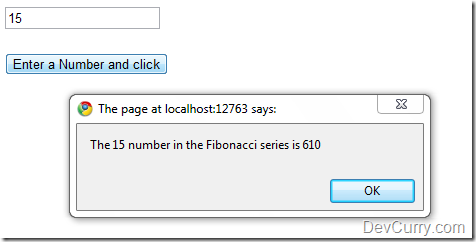# Find the Nth Number of Fibonacci series using JavaScript

 Tweet

Here’s some fun finding the Nth number of a Fibonacci series using JavaScript Recursion

`<html xmlns="http://www.w3.org/1999/xhtml" ><head><title>Nth Fibonacci Series</title><script type="text/javascript">    function callFibo() {        //extract textbox value        var num = document.getElementById('txtFibo').value;        //check if it is a number and greater than 1 and less than 36        if (isNaN(num)  ((num < 1)  (num > 35))) {            alert("Only numbers allowed. Enter a number > 0 and < 35");                    }        else {            alert("Number " + num + " in the Fibonacci series is " +                calcFiboNth(num));                           }    }    function calcFiboNth(num) {        if (num > 2) {            return calcFiboNth(num - 2) + calcFiboNth(num - 1);        } else {                         return 1;        }    }</script></head><body><input id="txtFibo" type="text" /><br /><br /><input id="btnFibo" type="button" value="Enter a Number and click"onClick="callFibo()" /></body></html>`

The function calcFiboNth() makes a recursive call to itself each time with a different ‘num’ value and this continues till ‘num’ reaches 1. That’s how you get the Nth number of the series.

Note: It is a good idea to add an upper limit to the number entered. Entering a higher number could take a very long time to compute the nth number of the Fibonacci series.See a Live Demo